Next: Three-Dimensional Quantum Mechanics Up: Identical Particles Previous: Identical Particles

### Exercises (N.B. Neglect spin in the following questions.)

1. Consider a system consisting of two non-interacting particles, and three one-particle states,,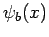, and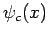. How many different two-particle states can be constructed if the particles are (a) distinguishable, (b) indistinguishable bosons, or (c) indistinguishable fermions?

2. Consider two non-interacting particles, each of mass, in a one-dimensional harmonic oscillator potential of classical oscillation frequency. If one particle is in the ground-state, and the other in the first excited state, calculate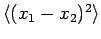assuming that the particles are (a) distinguishable, (b) indistinguishable bosons, or (c) indistinguishable fermions.

3. Two non-interacting particles, with the same mass, are in a one-dimensional box of length. What are the four lowest energies of the system? What are the degeneracies of these energies if the two particles are (a) distinguishable, (b) indistinguishable bosons, or (c) indistingishable fermions?

4. Two particles in a one-dimensional box of lengthoccupy the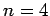and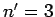states. Write the properly normalized wavefunctions if the particles are (a) distinguishable, (b) indistinguishable bosons, or (c) indistinguishable fermions.Next: Three-Dimensional Quantum Mechanics Up: Identical Particles Previous: Identical Particles
Richard Fitzpatrick 2010-07-20